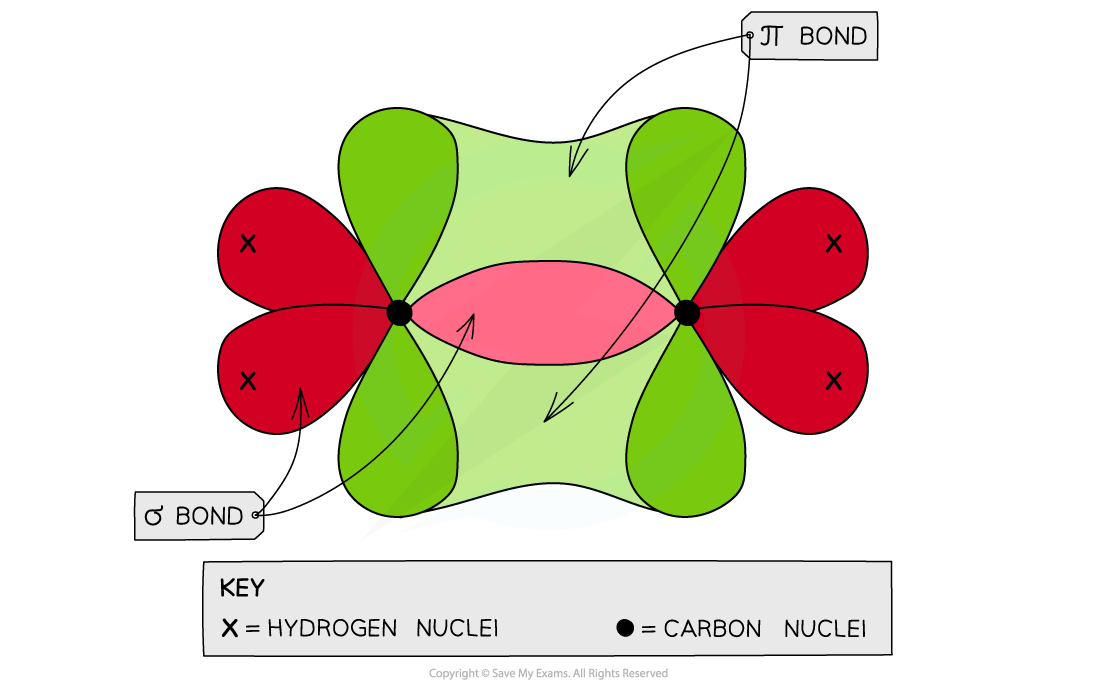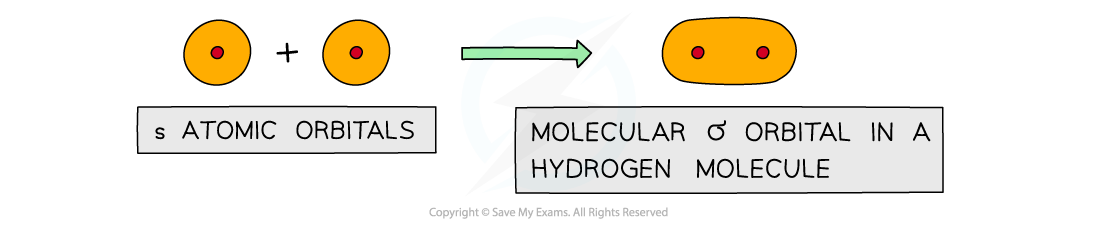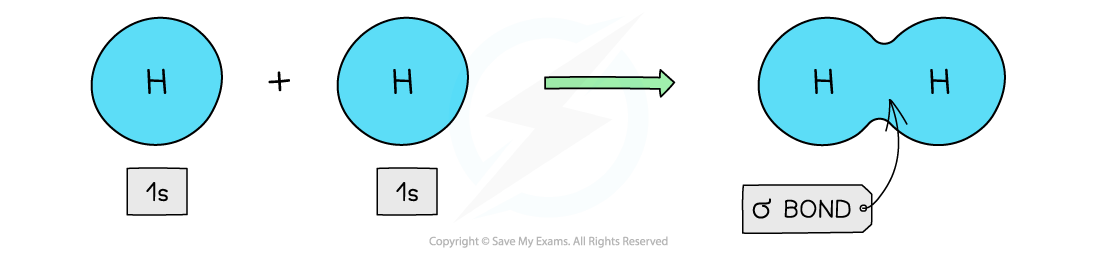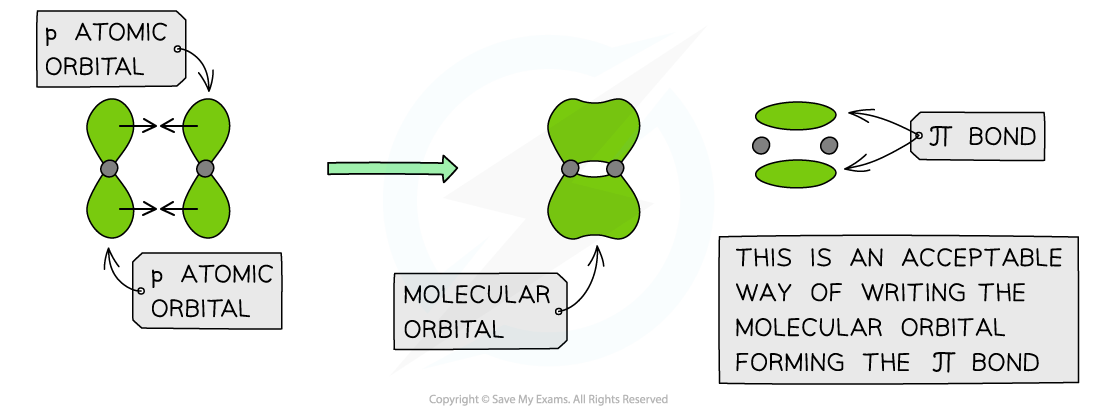# AQA A Level Chemistry复习笔记3.4.1 Structure, Bonding & Reactivity

### Shapes & Bond Angles in Organic Molecules

• Each carbon atom has four electrons in its outer shell (electronic configuration: 1s22s22p2)
• Carbon atoms share these four electrons in four covalent bonds with other atoms to achieve a full outer shell configuration
• These electrons are found in orbitals within the respective atoms
• When forming a covalent bond, the orbitals overlap in such a way to form two types of bonds
• Sigma bonds (σ)
• Pi bonds (π)

• When carbon atoms use only three of their electron pairs to form a σ bond, each carbon atom will have a p orbital which contains one spare electron
• When the p orbitals of two carbon atoms overlap with each other, a π bond is formed (the π bond contains two electrons)
• The two orbitals that form the π bond lie above and below the plane of the two carbon atoms to maximise bond overlap
• The three bonding pair of electrons are in the plane of the molecule and repel each other
• The molecule adopts a planar arrangement with bond angles of 120o

#### Ethene

• Each carbon atom uses three of its four electrons to form σ bonds
• Two σ bonds are formed with the hydrogen atoms
• One σ bond is formed with the other carbon atom
• The fourth electron from each carbon atom occupies a p orbital which overlaps sideways with another p orbital on the other carbon atom to form a π bond
• This means that the C-C is a double bond: one σ and one π bondThe overlap of the two p orbitals results in the formation of a π bond in ethene in which the bonding pair of electrons repel each other to force the molecule into a planar configuration with bond angles of 120 oEach carbon atom in ethene forms two sigma bonds with hydrogen atoms and one σ bond with another carbon atom. The fourth electron is used to form a π bond between the two carbon atoms

#### Exam Tip

A double bond is a combination of a σ and π bond and a triple bond is a combination of one σ and two π bonds. The strength of the bonds increases as follows: single < double < triple bond This is due to the increased electron density around the carbon-carbon bond, making the bond stronger and more difficult to break. You must be able to draw and explain these diagrams for the exam.

### Molecular Orbitals

#### Bond overlap in covalent bonds

• A single covalent bond is formed when two nonmetals combine
• Each atom that combines has an atomic orbital containing a single unpaired electron
• When a covalent bond is formed, the atomic orbitals overlap to form a combined orbital containing two electrons
• This new orbital is called the molecular orbital

• The greater the atomic orbital overlap, the stronger the bond
• Sigma (σ) bonds are formed by end-to-end overlap of orbitals between the bonding atoms
• Pi (π) bonds are formed by the sideways overlap of adjacent above and below the σ bond

#### σ bonds

• Sigma (σ) bonds are formed from the end to end overlap of atomic orbitals
• s orbitals overlap this way as well as p orbitalsSigma orbitals can be formed from the end to end overlap of s orbitals

• The electron density in a σ bond is symmetrical about a line joining the nuclei of the atoms forming the bond
• The pair of electrons is found between the nuclei of the two atoms
• The electrostatic attraction between the electrons and nuclei bonds the atoms to each other

#### Hydrogen

• The hydrogen atom has only one s orbital
• The s orbitals of the two hydrogen atoms will overlap to form a σ bond#### π bonds

• Pi (π) bonds are formed from the sideways overlap of adjacent p orbitals
• The two lobes that make up the π bond lie above and below the plane of the σ bond
• This maximises overlap of the p orbitals
• A single π bond is drawn as two electron clouds, one arising from each lobe of the p orbitals
• The two clouds of electrons in a π bond represent one bond containing two electronsπ orbitals can be formed from the sideways overlap of p orbitals